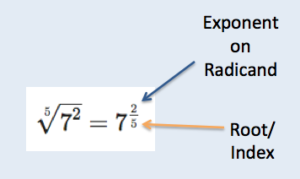## Radical Expressions and Rational Exponents

### Learning Outcomes

• Convert radicals to expressions with rational exponents
• Convert expressions with rational exponents to their radical equivalent

Square roots are most often written using a radical sign, like this, $\sqrt{4}$. But there is another way to represent them. You can use rational exponents instead of a radical. A rational exponent is an exponent that is a fraction. For example, $\sqrt{4}$ can be written as ${{4}^{\tfrac{1}{2}}}$.

Having difficulty imagining a number being raised to a rational power? They may be hard to get used to, but rational exponents can actually help simplify some problems. Writing radicals with rational exponents will come in handy when we discuss techniques for simplifying more complex radical expressions.

Radical expressions are expressions that contain radicals. Radical expressions come in many forms, from simple and familiar, such as$\sqrt{16}$, to quite complicated, as in $\sqrt{250{{x}^{4}}y}$.

## Write an Expression with a Rational Exponent as a Radical

Radicals and fractional exponents are alternate ways of expressing the same thing.  In the table below we show equivalent ways to express radicals: with a root, with a rational exponent, and as a principal root.

Exponent Form

Principal Root

$\sqrt{16}$ ${{16}^{\tfrac{1}{2}}}$ $4$
$\sqrt{25}$ ${{25}^{\tfrac{1}{2}}}$ $5$
$\sqrt{100}$ ${{100}^{\tfrac{1}{2}}}$ $10$

Let us look at some more examples, but this time with cube roots. Remember, cubing a number raises it to the power of three. Notice that in the examples in the table below, the denominator of the rational exponent is the number $3$.

Exponent Form

Principal Root

$\sqrt{8}$ ${{8}^{\tfrac{1}{3}}}$ $2$
$\sqrt{125}$ ${{125}^{\tfrac{1}{3}}}$ $5$
$\sqrt{1000}$ ${{1000}^{\tfrac{1}{3}}}$ $10$

These examples help us model a relationship between radicals and rational exponents: namely, that the nth root of a number can be written as either $\sqrt[n]{x}$ or ${{x}^{\frac{1}{n}}}$.

Exponent Form

$\sqrt{x}$ ${{x}^{\tfrac{1}{2}}}$
$\sqrt{x}$ ${{x}^{\tfrac{1}{3}}}$
$\sqrt{x}$ ${{x}^{\tfrac{1}{4}}}$
$\sqrt[n]{x}$ ${{x}^{\tfrac{1}{n}}}$

In the table above, notice how the denominator of the rational exponent determines the index of the root. So, an exponent of $\frac{1}{2}$ translates to the square root, an exponent of $\frac{1}{5}$ translates to the fifth root or $\sqrt{\quad}$, and $\frac{1}{8}$ translates to the eighth root or $\sqrt{\quad}$.

### Example

Express ${{(2x)}^{^{\frac{1}{3}}}}$ in radical form.

Remember that exponents only refer to the quantity immediately to their left unless a grouping symbol is used. The example below looks very similar to the previous example with one important difference—there are no parentheses! Look what happens.

### Example

Express $2{{x}^{^{\frac{1}{3}}}}$ in radical form.

## Write a Radical Expression as an Expression with a Rational ExponentFlexibility

We can write radicals with rational exponents, and as we will see when we simplify more complex radical expressions, this can make things easier. Having different ways to express and write algebraic expressions allows us to have flexibility in solving and simplifying them. It is like having a thesaurus when you write. You want to have options for expressing yourself!

### Example

Write $\sqrt{81}$ as an expression with a rational exponent.

### Example

Express $4\sqrt{xy}$ with rational exponents.

## Rational Exponents Whose Numerator is Not Equal to One

All of the numerators for the fractional exponents in the examples above were $1$. You can use fractional exponents that have numerators other than $1$ to express roots, as shown below.

Exponent

$\sqrt{9}$ $9^{\frac{1}{2}}$
$\sqrt{{{9}^{2}}}$ $9^{\frac{2}{3}}$
$\sqrt{9^{3}}$ $9^{\frac{3}{4}}$
$\sqrt{9^{2}}$ $9^{\frac{2}{5}}$
$\sqrt[n]{9^{x}}$ $9^{\frac{x}{n}}$To rewrite a radical using a fractional exponent, the power to which the radicand is raised becomes the numerator and the root/ index becomes the denominator.

### Writing Rational Exponents

Any radical in the form $\sqrt[n]{a^{x}}$  can be written using a fractional exponent in the form $a^{\frac{x}{n}}$.

The relationship between $\sqrt[n]{{{a}^{x}}}$and ${{a}^{\frac{x}{n}}}$ works for rational exponents that have a numerator of $1$ as well. For example, the radical $\sqrt{8}$ can also be written as $\sqrt{{{8}^{1}}}$, since any number remains the same value if it is raised to the first power. You can now see where the numerator of $1$ comes from in the equivalent form of ${{8}^{\frac{1}{3}}}$.

In the next example, we practice writing radicals with rational exponents where the numerator is not equal to one.

### Example

1. $\sqrt{{{a}^{6}}}$
2. $\sqrt{16^3}$

In our last example we will rewrite expressions with rational exponents as radicals. This practice will help us when we simplify more complicated radical expressions and as we learn how to solve radical equations. Typically it is easier to simplify when we use rational exponents, but this exercise is intended to help you understand how the numerator and denominator of the exponent are the exponent of a radicand and index of a radical.

### Example

Rewrite the expressions using a radical.

1. ${x}^{\frac{2}{3}}$
2. ${5}^{\frac{4}{7}}$

In the following video, we show more examples of writing radical expressions with rational exponents and expressions with rational exponents as radical expressions.

We will use this notation later, so come back for practice if you forget how to write a radical with a rational exponent.

## Summary

Any radical in the form $\sqrt[n]{a^{x}}$ can be written using a fractional exponent in the form $a^{\frac{x}{n}}$. Rewriting radicals using fractional exponents can be useful when simplifying some radical expressions. When working with fractional exponents, remember that fractional exponents are subject to all of the same rules as other exponents when they appear in algebraic expressions.